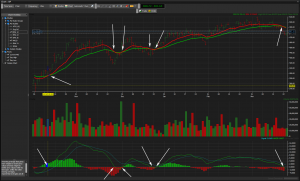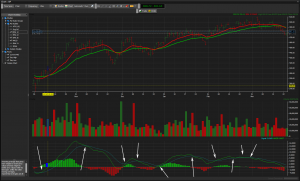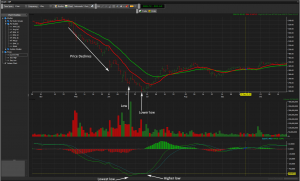MACD or Moving Average Convergence Divergence is a momentum indicator. It is formed by taking two moving averages, typically the 12 day and 26 day exponential moving averages(EMA) and subtracting the value of longer moving average from the shorter one, so this gives the MACD line itself. This turns two trend following indicators, the moving averages, into a momentum oscillator which is what make MACD so popular.

There is also another value required to make signal line of the MACD indicator. The signal line is just an EMA of the MACD line itself and the value that is most commonly used is 9. Some simple strategies for trading the MACD are when it crosses the signal line, when it crosses the centre line(zero) or divergences of the MACD line and price action.

The MACD is not overlaid on price but is displayed independently, usually below the price chart. Included in the MACD chart is a histogram that is calculated by subtracting the MACD value from the signal line value.

## Simple MACD Strategies

### Centreline Crossover

When the MACD line crosses the centre line it can present a trade in the direction that the cross takes place. The important thing to remember about a centre line cross of the MACD is that it represents a cross of the two moving averages that are used in forming the MACD line. Therefore do not get excited and think you are seeing two indications to make the same trade as the moving average cross and the MACD centre line cross are indeed the same thing.

Lets take look at a simple example:Chart provided courtesy of SpreadCo, who have some of the tightest spreads available.
Chart Key:
Thick Red line on Price = 12 EMA
Thick green line on Price = 26 EMA
Dashed blue line on MACD = Signal line (9 EMA of MACD)
Thin green line on MACD = MACD
Green Histogram bars= days when

As you can see I have highlighted the points at which the MACD crosses the centreline and also where the two moving averages cross.

If you were to trade this strategy only buying when the MACD crosses up passed the centreline you would have made three trades:
Sell at 416
2 points profit

Sell at 432
31 points loss

Sell at 474
16 points profit

Therefore overall you would have made 2 – 31 + 16 = -13 therefore a 13 point loss.
Obviously you don’t have to only trade long you can sell short via a spread betting or CFD account.

### Signal Line Crossover

When the MACD crosses the signal line it can be a signal to trade in the direction of the cross. For instance if the MACD crosses up through the signal line then this would be classed as a buy signal and when it crosses down through the MACD this is a sell signal.

Lets take a look at a simple example:Chart provided courtesy of SpreadCo, who have some of the tightest spreads available.

Sell 363
27 points loss

Sell 442
39 points profit

Sell 446
20 points loss

Sell 466
5 points profit

Sell 483
7 points loss

Then we face several buy and sell signals in quick succession. This known as whipsaws, where you buy and sell quickly usually making a loss. These whipsaw losses are what reduces the appeal of trading such strategies.

This example would have made -27 + 39 – 20 +5 – 7 = -10 therefore a 10 point loss. Again you do not need to always trade long you can trade short or even both ways.

### MACD/Price Divergence

The MACD/Price divergence strategy is when the instrument price makes a new low and the MACD makes a higher low. This is known as a bullish divergence and would signal a long trade. The converse is true for a short trading signal.

Lets take a look at a simple example:Chart provided courtesy of SpreadCo, who have some of the tightest spreads available.

This would have give you a buy signal in June 2010. While this gives you an entry signal it does not tell you when to exit the trade, therefore this needs to be considered before making a trade. While this example shows a good divergence caution should be used as many false signals can arise. Using MACD/Price divergence as part of a wider strategy can sometimes be useful.